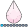## SGPLOT VBOX - Category Order

Hi All,

I am trying to complete a boxplot, but the group order in figure is not what I want. As in the figure below, I want the group order is E9.5, E10.5, E11.5. And here is my code:

proc sgplot data=lab;
vbox lin__1 / category=group group=group gropdisplay=cluster
lineattrs=(pattern=solid) whiskerattrs=(pattern=solid);
xaxis display=(nolabel);
yaxis grid;
run;

Any way can change it?

Thanks,

Chen3 REPLIES 3

## Re: SGPLOT VBOX - Category Order

Lots of options, but a simple way is to sort the data in the data set and then use

the GROUPORDER=DATA option on the VBOX statement.

## Re: SGPLOT VBOX - Category Order

Another option is to use CATEGORY= instead of GROUP=.  Then you can set the values by using the XAXIS statement, as this example shows:

``````
proc sgplot data=sashelp.cars;
vbox mpg_city / category=origin;
xaxis type=discrete values=('USA' 'Asia' 'Europe');
run;``````djrisks
Barite | Level 11

## Re: SGPLOT VBOX - Category Order

Hi Chen,

You can try out the grouporder option within vbox. Another options that will definitely work is to create a format based on the variable group. So you can create a numeric column based on group, i.e. groupn, and that will have the values, 1, 2 and 3. Then you can use that numeric variable in the plot: i.e.

proc format;

value grouporder 1 = "E9.5"

2 = "E10.5"

3 = "E11.5";

run;

proc sort data = lab;

by groupn;

run;

proc sgplot data=lab;
vbox lin__1 / category=groupn group=groupn gropdisplay=cluster
lineattrs=(pattern=solid) whiskerattrs=(pattern=solid);
xaxis display=(nolabel);
yaxis grid;

format groupn grouporder.;
run;

Discussion stats
• 3 replies
• 9327 views
• 1 like
• 3 in conversation